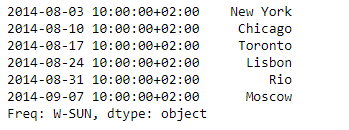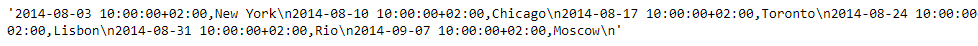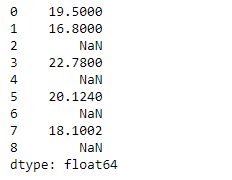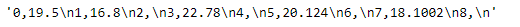Open in App
Not now

# Python | Pandas Series.to_csv()

• Difficulty Level : Expert
• Last Updated : 24 Jun, 2020

Pandas series is a One-dimensional ndarray with axis labels. The labels need not be unique but must be a hashable type. The object supports both integer- and label-based indexing and provides a host of methods for performing operations involving the index.

Pandas` Series.to_csv()` function write the given series object to a comma-separated values (csv) file/format.

Syntax: Series.to_csv(*args, **kwargs)

Parameter :
path_or_buf : File path or object, if None is provided the result is returned as a string.
sep : String of length 1. Field delimiter for the output file.
na_rep : Missing data representation.
float_format : Format string for floating point numbers.
columns : Columns to write
header : If a list of strings is given it is assumed to be aliases for the column names.
index : Write row names (index).
index_label : Column label for index column(s) if desired. If None is given, and header and index are True, then the index names are used.
mode : Python write mode, default ‘w’.
encoding : A string representing the encoding to use in the output file.
compression : Compression mode among the following possible values: {‘infer’, ‘gzip’, ‘bz2’, ‘zip’, ‘xz’, None}.
quoting : Defaults to csv.QUOTE_MINIMAL.
quotechar : String of length 1. Character used to quote fields.

Returns : None or str

Example #1: Use `Series.to_csv()` function to convert the given series object to csv format.

 `# importing pandas as pd ` `import` `pandas as pd ` ` `  `# Creating the Series ` `sr ``=` `pd.Series([``'New York'``, ``'Chicago'``, ``'Toronto'``, ``'Lisbon'``, ``'Rio'``, ``'Moscow'``]) ` ` `  `# Create the Datetime Index ` `didx ``=` `pd.DatetimeIndex(start ``=``'2014-08-01 10:00'``, freq ``=``'W'``,  ` `                     ``periods ``=` `6``, tz ``=` `'Europe / Berlin'``)  ` ` `  `# set the index ` `sr.index ``=` `didx ` ` `  `# Print the series ` `print``(sr) `

Output :Now we will use `Series.to_csv()` function to convert the given Series object into a comma separated format.

 `# convert to comma-separated ` `sr.to_csv() `

Output :As we can see in the output, the `Series.to_csv()` function has converted the given Series object into a comma-separated format.

Example #2: Use `Series.to_csv()` function to convert the given series object to csv format.

 `# importing pandas as pd ` `import` `pandas as pd ` ` `  `# Creating the Series ` `sr ``=` `pd.Series([``19.5``, ``16.8``, ``None``, ``22.78``, ``None``, ``20.124``, ``None``, ``18.1002``, ``None``]) ` ` `  `# Print the series ` `print``(sr) `

Output :Now we will use `Series.to_csv()` function to convert the given Series object into a comma separated format.

 `# convert to comma-separated ` `sr.to_csv() `

Output :As we can see in the output, the `Series.to_csv()` function has converted the given Series object into a comma-separated format.

My Personal Notes arrow_drop_up
Related Articles